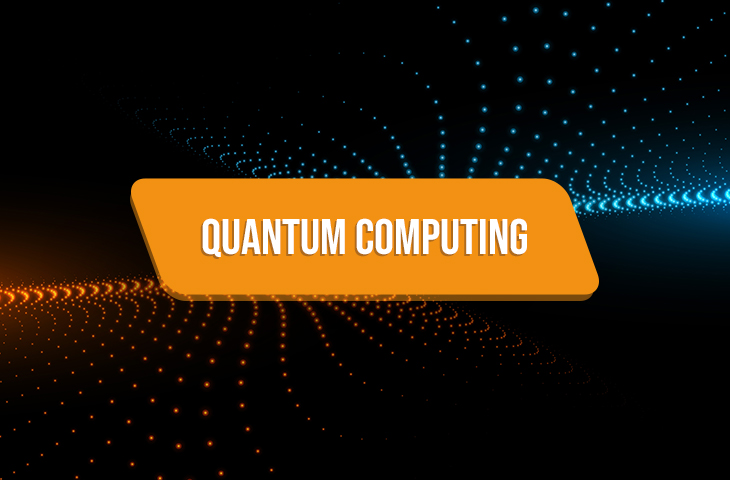# POSTS## What is Quantum Computing (Supercomputing)? How do Quantum Computing work?

The word Quantum itself is the representation of some sort of computing power. Also the Quantum Computing never goes away from the headlines.

Quantum Computing also the other name of supercomputing is very necessary in today’s time. It is used to predict so many things and it has end number of uses – hence the Supercomputers cannot be replaced now. Here in this article we are going to discuss about – Quantum Computing also known as Supercomputing, it's history (the saga), it’s definition, understanding the basic concept of Quantum Computing and also how Quantum Computing works.

THE SAGA OF QUANTUM COMPUTING:

Quantum Computing began in the year (early) 1980s. The first ever model of Quantum Computing was suggested by the physicist Paul Benioff. He suggested the model of the Turing Machine. Later Richard Feynman and Yuri Main suggested that a quantum computer could perform simulations that are out of reach for regular computers. Then later in the year 1994, Peter Shor developed a polynomial-time quantum algorithm for factoring integers. Then, they experimented to develop a quantum computer.

DEFINITION OF QUANTUM COMPUTING:

You have learnt the history of Quantum Computing but do you exactly know what the definition of Quantum Computing is? If no – then here it is. Quantum computing puts it’s main focus on developing computer technology based on the principles of Quantum Theory. The quantum theory explains the behavior of energy and material on the atomic and subatomic levels.

The computers we use normally are classical computers, that is the computers we use can only process data of value ranging from 0 to 10 and due to this the ability of the computers remain restricted, whereas the quantum computers process data in bits and qubits, this allows them to harness the unique ability of subatomic participles that allows them to exist in more than one state i.e. a 1 and a 0 at the same time.

Quantum computers can also use the other aspect quantum mechanics that is entanglement – but this aspect has a negative point that is – if one tries to look at the sub – atomic particles then that person could end up bumping them and thus their values can change.

To develop a practical quantum computer, scientists have to devise ways of making measurements indirectly to preserve the system's integrity. On one hand the classical computers are good at calculus, the quantum computer is even better at sorting, finding prime numbers, simulating molecules, and optimization, and thus could open the door to a new computing era,” a Morgan Stanley report noted.

THE WORKING OF QUANTUM COMPUTING:

In quantum computing the memory is processed in bits. Also we can measure the "size" of the memory in terms of the number of bits needed to represent fully the state of the memory.

If the memory of the quantum computer follows the rules or the laws of quantum physics, the state of the memory could be in a quantum superposition of different possible "classical" states. One of the most significant property of Quantum computers is that they have the ability to turn classical memory states into quantum memory states, and the vice-versa of it.

As we can only observe classical States it becomes the duty of the quantum computers to return to us the classical outputs and to produce to us the classical output the quantum computers will have to measure the various size of the memory at various times throughout the computation process.

What happens in quantum mechanics, is that the, probability vectors are generalized to density operators. In this we focus only on the quantum state vector formalism.

One can easily represent any given quantum computation as a network of quantum logic gates from a fairly small family of gates.

CONCLUSION:

Here in this article, you have learnt about the Quantum Computing. You all know how necessary Quantum computing has become now. Quantum Computing can perform many such function which currently do not have any replacement. Quantum computing serves so many purposes which if not fulfilled we may find ourselves stuck. It is quantum computing which helps us to track the upcoming storms and other disasters.